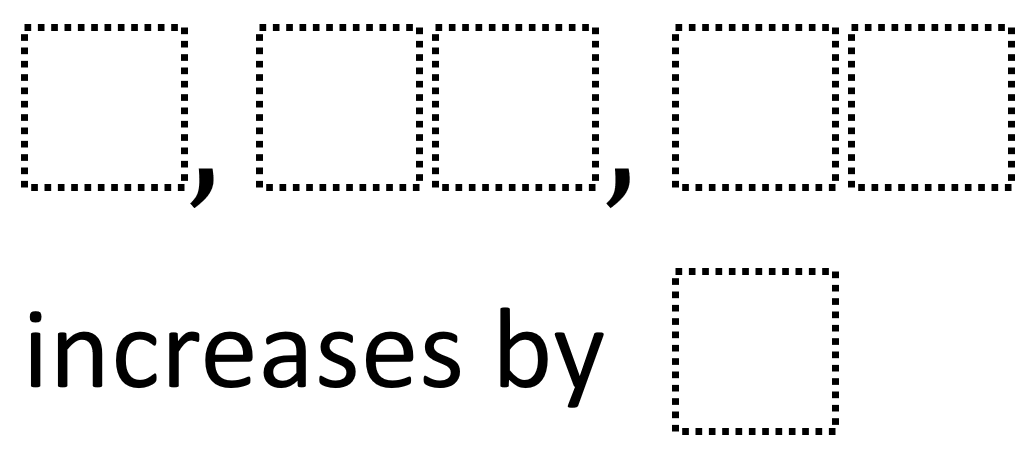# Simple Patterns 1

Directions: Using the digits 1 to 9 at most one time each, place a digit in each box to make a true pattern.### Hint

How can we tell whether the digits we place in the boxes actually make a pattern? How can we tell if the pattern increases each time by the digit we choose? How can we tell what would be a bad digit to choose for how much it increases by?

There are many possible answers including 8, 17, 26 increases by 9.

Source: Robert Kaplinsky

## Multi Digit Division 3

Directions: Using the digits 1 to 9 at most one time each, place a digit …

1.2.